Open In App

# How to select rows from a dataframe based on column values ?

Prerequisite: Pandas.Dataframes in Python

In this article, we will cover how we select rows from a DataFrame based on column values in Python

The rows of a Dataframe can be selected based on conditions as we do use the SQL queries. The various methods to achieve this is explained in this article with examples.

## Importing Dataset for demonstration

To explain the method a dataset has been created which contains data of points scored by 10 people in various games. The dataset is loaded into the Dataframe and visualized first. Ten people with unique player id(Pid) have played different games with different game id(game_id) and the points scored in each game are added as an entry to the table. Some of the player’s points are not recorded and thus NaN value appears in the table.

## Python3

 `import` `pandas as pd` `df ``=` `pd.read_csv(r``"__your file path__\example2.csv"``)``print``(df)`

Output: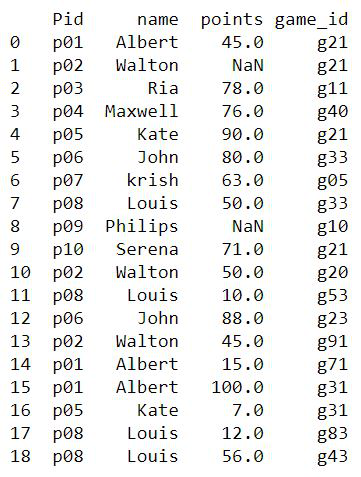dataset example2.csv

We will select rows from Dataframe based on column value using:

• Boolean Indexing method
• Positional indexing method
• Using isin() method
• Using Numpy.where() method
• Comparison with other methods

## Method 1: Boolean Indexing method

In this method, for a specified column condition, each row is checked for true/false. The rows which yield True will be considered for the output. This can be achieved in various ways. The query used is Select rows where the column Pid=’p01′

### Example 1: Select rows from a Pandas DataFrame based on values in a column

In this example, we are trying to select those rows that have the value p01 in their column using the equality operator.

## Python3

 `# Choose entries with id p01``df_new ``=` `df[df[``'Pid'``] ``=``=` `'p01'``]` `print``(df_new)`

Output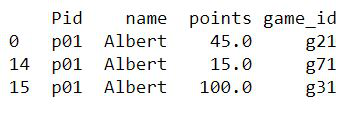### Example 2: Specifying thecondition ‘mask’ variable

Here, we will see Pandas select rows by condition the selected rows are assigned to a new Dataframe with the index of rows from the old Dataframe as an index in the new one and the columns remaining the same.

## Python3

 `# condition mask``mask ``=` `df[``'Pid'``] ``=``=` `'p01'` `# new dataframe with selected rows``df_new ``=` `pd.DataFrame(df[mask])` `print``(df_new)`

Output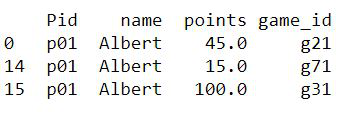### Example 3: Combining mask and dataframes.values property

The query here is to Select the rows with game_id ‘g21’.

## Python3

 `# condition with df.values property``mask ``=` `df[``'game_id'``].values ``=``=` `'g21'` `# new dataframe``df_new ``=` `df[mask]` `print``(df_new)`

Output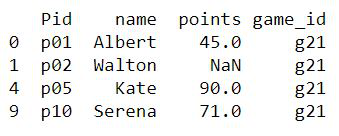## Method 2: Positional indexing method

The methods loc() and iloc() can be used for slicing the Dataframes in Python. Among the differences between loc() and iloc(), the important thing to be noted is iloc() takes only integer indices, while loc() can take up boolean indices also.

### Example 1: Pandas select rows by loc() method based on column values

The mask gives the boolean value as an index for each row and whichever rows evaluate to true will appear in the result. Here, the query is to select the rows where game_id is g21.

## Python3

 `# for boolean indexing``mask ``=` `df[``'game_id'``].values ``=``=` `'g21'` `# using loc() method``df_new ``=` `df.loc[mask]` `print``(df_new)`

Output### Example 2: Pandas select rows by iloc() method based on column values

The query is the same as the one taken above. The iloc() takes only integers as an argument and thus, the mask array is passed as a parameter to the Numpy’s flatnonzero() function that returns the index in the list where the value is not zero (false)

## Python3

 `# condition mask``mask ``=` `df[``'game_id'``].values ``=``=` `'g21'``print``(``"Mask array :"``, mask)` `# getting non zero indices``pos ``=` `np.flatnonzero(mask)``print``(``"\nRows selected :"``, pos)` `# selecting rows``df.iloc[pos]`

Output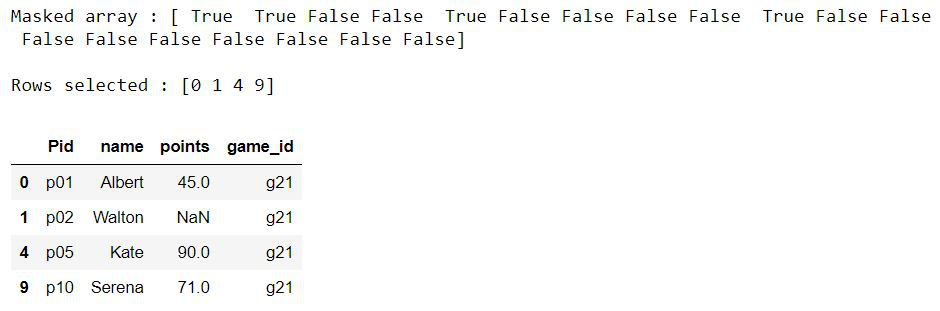## Method 3: Using dataframe.query() method

The query() method takes up the expression that returns a boolean value, processes all the rows in the Dataframe, and returns the resultant Dataframe with selected rows.

### Example 1: Pandas select rows by Dataframe.query() method based on column values

Select  rows where the name=”Albert”

## Python3

 `df.query(``'name=="Albert"'``)`

Output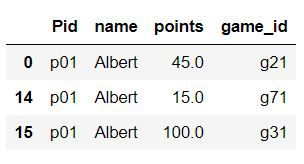### Example 2: Select rows based on iple column conditions

This example is to demonstrate that logical operators like AND/OR can be used to check multiple conditions. we are trying to select rows where points>50 and the player is not Albert.

## Python3

 `df.query(``'points>50 & name!="Albert"'``)`

Output## Method 3: Using isin() method

This method of Dataframe takes up an iterable or a series or another Dataframe as a parameter and checks whether elements of the Dataframe exist in it. The rows that evaluate to true are considered for the resultant.

### Select rows whose column value is in an iterable array

Select the rows where players are Albert, Louis, and John.

## Python3

 `# Players to be selected``li ``=` `[``'Albert'``, ``'Louis'``, ``'John'``]` `df[df.name.isin(li)]`

Output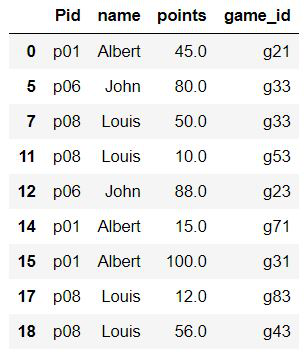### Example 2: Select rows where the column does not equal a value

The tiled symbol (~) provides the negation of the expression evaluated. Here, we are selecting rows where points>50 and players are not Albert, Louis, and John.

## Python3

 `# values to be present in selected rows``li ``=` `[``'Albert'``, ``'Louis'``, ``'John'``]` `# selecting rows from dataframe``df[(df.points > ``50``) & (~df.name.isin(li))]`

Output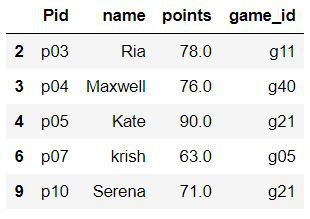## Method 4: Using Numpy.where() method

The Numpy’s where() function can be combined with the pandas’ isin() function to produce a faster result. The numpy.where() is proved to produce results faster than the normal methods used above.

## Python3

 `import` `numpy as np` `df_new ``=` `df.iloc[np.where(df.name.isin(li))]`

Output: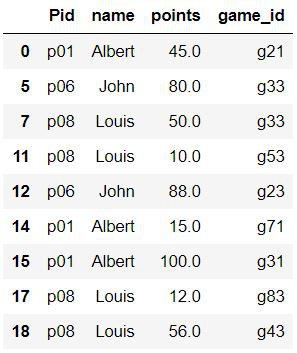## Method 5: Comparison with other methods

### Example 1

In this example, we are using a mixture of NumPy and pandas method

## Python3

 `# to calculate timing``import` `numpy as np``%` `%` `timeit`  `# using mixture of numpy and pandas method``df_new ``=` `df.iloc[np.where(df.name.isin(li))]`

Output:

756 µs ± 132 µs per loop (mean ± std. dev. of 7 runs, 1000 loops each)

### Example 2

In this example, we are using only the Pandas method

## Python3

 `# to calculate time``%``%``timeit` `li``=``[``'Albert'``,``'Louis'``,``'John'``]` `# Pandas method only``df[(df.points>``50``)&(~df.name.isin(li))]`

Output

1.7 ms ± 307 µs per loop (mean ± std. dev. of 7 runs, 1000 loops each)